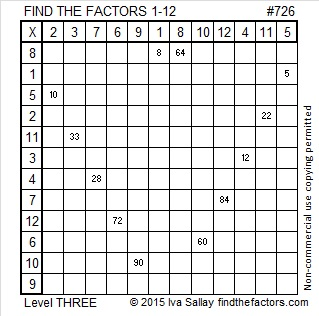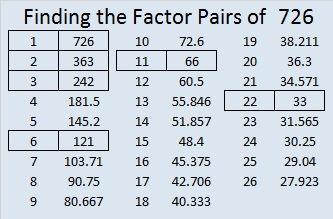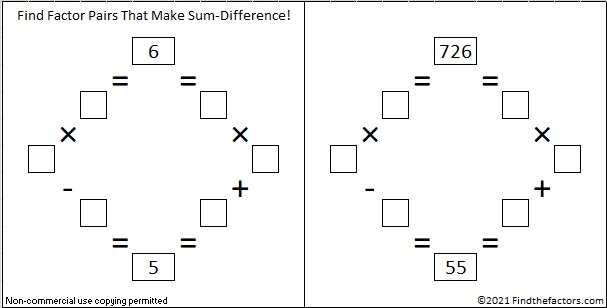# Simplifying √726

Contents

### Today’s Puzzle:

This level 3 puzzle is not difficult to do. If you start at the top of the first column and work down cell by cell, you’ll catch the rhythm of finding all the factors that can make this puzzle function as a multiplication table:Print the puzzles or type the solution on this excel file: 12 Factors 2015-12-28### Simplifying √726:

Most numbers whose square roots can be simplified are divisible by 4, 9 or both. If I were trying to simplify √726, I would first apply divisibility tricks for 4 and 9 to the number 726:

• 726 is even so it is divisible by 2, but since 26 is not divisible by 4, neither is 726.
• Also notice that 7 + 2 + 6 = 15, a number divisible by 3, but not by 9. That means 726 is divisible by 3, but not by 9.

Thus 726 is NOT divisible by either 4 or 9. I will make a little cake to see if it is divisible by any other square numbers.

Dividing by 2 and then by 3 could be a good place to start, but rather than do those two divisions, I would simply do one division as I divide 726 by 6:I would immediately recognize that 121 is 11 × 11, so I don’t need to make any more layers for this factoring cake!

To simplify √726, take the square root of everything on the outside of this cake (the numbers in red): Thus, √726 = (√6)(√121) = 11√6.

### Factors of 726:

• 726 is a composite number.
• Prime factorization: 726 = 2 x 3 x 11 x 11, which can be written 726 = 2 x 3 x 11²
• The exponents in the prime factorization are 1, 1, and 2. Adding one to each and multiplying we get (1 + 1)(1 + 1)(2 + 1) = 2 x 2 x 3 = 12. Therefore 726 has exactly 12 factors.
• Factors of 726: 1, 2, 3, 6, 11, 22, 33, 66, 121, 242, 363, 726
• Factor pairs: 726 = 1 x 726, 2 x 363, 3 x 242, 6 x 121, 11 x 66, or 22 x 33
• Taking the factor pair with the largest square number factor, we get √726 = (√121)(√6) = 11√6 ≈ 26.944387.### Sum-Difference Puzzles:

6 has two factor pairs. One of those pairs adds up to 5, and the other one subtracts to 5. Put the factors in the appropriate boxes in the first puzzle.

726 has six factor pairs. One of the factor pairs adds up to ­55, and a different one subtracts to 55. If you can identify those factor pairs, then you can solve the second puzzle!The second puzzle is really just the first puzzle in disguise. Why would I say that?

### More Facts about the Number 726:

726 is the average of 11² and 11³ which is a characteristic that also makes it the 11th Pentagonal pyramidal number.

{724, 725, 726} is only the second set of three consecutive numbers in which each number in the set is the product of exactly one number squared and one different number. {603, 604, 605} was the first set.

726 is the sum of consecutive prime numbers 359 and 367.

726 is a palindrome in three bases:

• 10401 BASE 5; note that 1(625) + 0(125) + 4(25) + 0(5) + 1(1) = 726.
• 141 BASE 25; note that 1(625) + 4(25) + 1(1) = 726.
• MM BASE 32 (M = 22 base 10); note that 22(32) + 22(1) = 726.

## 4 thoughts on “Simplifying √726”

1.Paula Beardell Krieg

“726 is a palindrome in three bases:” Reading this makes me happy.

•ivasallay

I’m so glad you told me that. Sometimes I’ve wondered if finding palindromes in other bases was just one way to be weird.

•Paula Beardell Krieg

It’s so weird it’s wonderful..just like publishing Find the Factors everyday. There is so much to thank you for.

•ivasallay

Thank you. I guess we are all unique and weird in our own way, and the world is better for it.

This site uses Akismet to reduce spam. Learn how your comment data is processed.